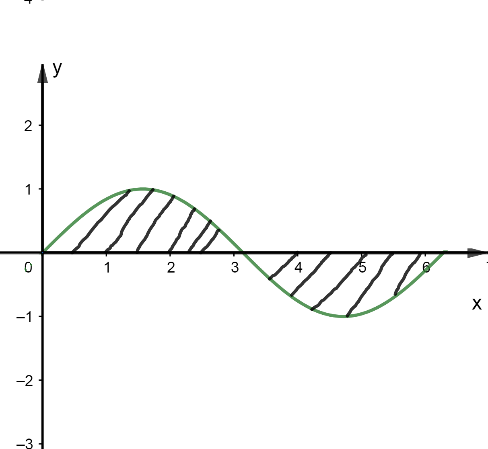Courses
Courses for Kids
Free study material
Free LIVE classes
MoreLIVE
Join Vedantu’s FREE Mastercalss

# The maximum value of $4{{\sin }^{2}}x-12\sin x+7$ is.A. 25B. 4C. Does not existD. None of theseVerified
360.6k+ views
Hint: We know that the value of $\sin \theta$ varies from – 1 to 1. So, from this concept we will try to find the maximum value of the given equation.

Complete step-by-step Solution:
It is given in the question that we have to find the maximum value of,
$\Rightarrow 4{{\sin }^{2}}x-12\sin x+7$
Taking 4 common from $4{{\sin }^{2}}x-12\sin x$, we get,
$\Rightarrow 4\left( {{\sin }^{2}}x-3\sin x \right)+7$
Now, we will add and subtract ${{\left( \dfrac{3}{2} \right)}^{2}}$ and also multiply and divide the term $3\sin x$ by 2. So, we will get,
$\Rightarrow 4\left[ {{\sin }^{2}}x-2.\sin x.\dfrac{3}{2}+{{\left( \dfrac{3}{2} \right)}^{2}}-{{\left( \dfrac{3}{2} \right)}^{2}} \right]+7$
Since we know that ${{\left( a+b \right)}^{2}}={{a}^{2}}+2ab+{{b}^{2}}$ , we write the above equation as,
$\Rightarrow 4\left[ {{\left( \sin x-\dfrac{3}{2} \right)}^{2}}-\dfrac{9}{4} \right]+7$
On opening the bracket and multiplying the terms inside the bracket with 4, we get,
\begin{align} & \Rightarrow 4{{\left( \sin x-\dfrac{3}{2} \right)}^{2}}-\dfrac{9}{4}\times 4+7 \\ & \Rightarrow 4{{\left( \sin x-\dfrac{3}{2} \right)}^{2}}-9+7 \\ & \Rightarrow 4{{\left( \sin x-\dfrac{3}{2} \right)}^{2}}-2 \\ \end{align}
We know that, the value of sin varies from – 1 to 1
$\Rightarrow -1\le \sin x\le 1..........\left( 1 \right)$(Graph of sinx)
Subtracting $\dfrac{3}{2}$ from all sides in equation (1), we get,
\begin{align} & \Rightarrow -1-\dfrac{3}{2}\le \left( \sin x-\dfrac{3}{2} \right)\le +1-\dfrac{3}{2} \\ & or \\ & \Rightarrow \dfrac{-5}{2}\le \left( \sin x-\dfrac{3}{2} \right)\le \dfrac{-1}{2} \\ \end{align}
On squaring all sides fraction sign changes, we get,
$\Rightarrow \dfrac{1}{4}\le {{\left( \sin x-\dfrac{3}{2} \right)}^{2}}\le \dfrac{25}{4}$
On multiplying the above expression by 4, we get,
$\Rightarrow 1\le 4{{\left( \sin x-\dfrac{3}{2} \right)}^{2}}\le 25$
Subtracting 2 from all sides, we get,
\begin{align} & \Rightarrow 1-2\le 4{{\left( \sin x-\dfrac{3}{2} \right)}^{2}}-2\le 25-2 \\ & \Rightarrow -1\le 4{{\left( \sin x-\dfrac{3}{2} \right)}^{2}}-2\le 23 \\ \end{align}
Hence, the maximum value of $\left[ 4{{\left( \sin x-\dfrac{3}{2} \right)}^{2}}-2 \right]\ \ is\ \ 23$.
Or the maximum value of $4{{\sin }^{2}}x-12\sin x+7$ is 23.
Therefore option (D) is the correct answer.

Note: This question can be solved in just a few steps. You just need to memorise the graph of sin x and keeping limits of sin in mind to solve this problem. This will save your time.
One mistake that can be committed is by not changing the inequality sign and getting the wrong result.
Last updated date: 27th Sep 2023
Total views: 360.6k
Views today: 9.60k